Journal topic
Atmos. Meas. Tech., 12, 1861–1870, 2019
https://doi.org/10.5194/amt-12-1861-2019Atmos. Meas. Tech., 12, 1861–1870, 2019
https://doi.org/10.5194/amt-12-1861-2019Research article 20 Mar 2019

Research article | 20 Mar 2019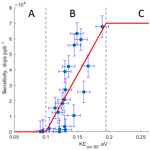# Using collision-induced dissociation to constrain sensitivity of ammonia chemical ionization mass spectrometry (NH4+ CIMS) to oxygenated volatile organic compounds

Using collision-induced dissociation to constrain sensitivity of ammonia chemical ionization mass spectrometry (NH4+ CIMS) to oxygenated volatile organic compounds
Alexander Zaytsev1, Martin Breitenlechner1, Abigail R. Koss2, Christopher Y. Lim2, James C. Rowe2, Jesse H. Kroll2, and Frank N. Keutsch1,3,4 Alexander Zaytsev et al.
• 1John A. Paulson School of Engineering and Applied Sciences, Harvard University, Cambridge, MA 02138, USA
• 2Department of Civil and Environmental Engineering, Massachusetts Institute of Technology, Cambridge, MA 02139, USA
• 3Department of Chemistry and Chemical Biology, Harvard University, Cambridge, MA 02138, USA
• 4Department of Earth and Planetary Sciences, Harvard University, Cambridge, MA 02138, USA

Correspondence: Alexander Zaytsev (zaytsev@g.harvard.edu)

Abstract

Chemical ionization mass spectrometry (CIMS) instruments routinely detect hundreds of oxidized organic compounds in the atmosphere. A major limitation of these instruments is the uncertainty in their sensitivity to many of the detected ions. We describe the development of a new high-resolution time-of-flight chemical ionization mass spectrometer that operates in one of two ionization modes: using either ammonium ion ligand-switching reactions such as for ${\mathrm{NH}}_{\mathrm{4}}^{+}$ CIMS or proton transfer reactions such as for proton-transfer-reaction mass spectrometer (PTR-MS). Switching between the modes can be done within 2 min. The ${\mathrm{NH}}_{\mathrm{4}}^{+}$ CIMS mode of the new instrument has sensitivities of up to 67 000 dcps ppbv−1 (duty-cycle-corrected ion counts per second per part per billion by volume) and detection limits between 1 and 60 pptv at 2σ for a 1 s integration time for numerous oxygenated volatile organic compounds. We present a mass spectrometric voltage scanning procedure based on collision-induced dissociation that allows us to determine the stability of ammonium-organic ions detected by the ${\mathrm{NH}}_{\mathrm{4}}^{+}$ CIMS instrument. Using this procedure, we can effectively constrain the sensitivity of the ammonia chemical ionization mass spectrometer to a wide range of detected oxidized volatile organic compounds for which no calibration standards exist. We demonstrate the application of this procedure by quantifying the composition of secondary organic aerosols in a series of laboratory experiments.

1 Introduction

Understanding the photochemical oxidation of volatile organic compounds (VOCs) in the atmosphere is crucial for estimating their contribution to the formation of secondary organic aerosol (SOA) and tropospheric ozone, key components of photochemical smog (Atkinson, 2000; Shrivastava et al., 2017). Identification and quantification of VOCs have remained an analytical challenge due to the complexity of multigenerational chemical systems and high variability in VOC concentrations in the atmosphere.

Chemical ionization mass spectrometry (CIMS) has become an important analytical tool for measurements of organic molecules in the atmosphere. Reagent ions are typically produced by glow discharge (Hansel et al., 1995) or a radioactive ion source (Blake et al., 2004). These ions subsequently react with analyte molecules by ligand switching, reactive electron transfer, or proton transfer and form product ions which are later detected by a mass spectrometer. Many modern CIMS instruments use time-of-flight mass spectrometers (ToF-MSs) which have high mass resolving power and simultaneous detection of all ions. Some of the benefits of CIMS instruments include high sensitivity, fast time response, linearity, and reproducibility. A variety of reagent ions can be used to detect different classes of VOCs. Nitrate ion CIMS has been used to detect highly oxidized organic molecules as well as sulfuric acid (Berresheim et al., 2000; Jokinen et al., 2012). Iodide adduct CIMS and acetate CIMS (both negative ion polarity) have played a key role in the measurement of carboxylic acids (Lee et al., 2014; Bertram et al., 2011). CF3O CIMS has been used to measure specific classes of VOCs such as hydroperoxides (Crounse et al., 2006). Protonated water clusters have been used to detect a broad range of chemical compounds containing oxygen, nitrogen, and sulfur (Lindinger et al., 1998; Yuan et al., 2017). Recently, two new proton-transfer-reaction time-of-flight mass spectrometers have been developed: the PTR3 (Breitenlechner et al., 2017) and the VOCUS PTR-TOF (Krechmer et al., 2018). Using H3O+ reagent ions, both instruments show sensitivities exceeding 10 000 cps ppbv−1 (counts per second per part per billion by volume) for select compounds. Detection efficiency and sensitivity of CIMS instruments depend critically on both the reagent ion and the measured sample molecule (Hyttinen et al., 2017).

CIMS instruments have also been used for analyzing submicrometer particulate organic matter. Hellen et al. (2008) equipped the inlet of the proton-transfer-reaction mass spectrometer (PTR-MS) instrument with a denuder to remove the gas-phase organics and a heater to vaporize the aerosol particles. Similarly, Eichler et al. (2015) introduced the CHARON–PTR-ToF-MS setup that transmits particles with a 75 %–90 % efficiency. FIGAERO–HRToF-CIMS (Lopez-Hilfiker et al., 2014) uses a new filter inlet for thermal desorption of ambient submicron particles.

In this work, we describe the use of protonated ammonia molecules (ammonium, ${\mathrm{NH}}_{\mathrm{4}}^{+}$) for soft ionization of analyte molecules. Ammonium has been previously used as a CIMS reagent ion. Lindinger et al. (1998) showed that proton transfer reactions can be utilized to softly ionize VOCs, yielding product ions VOCH+:

$\begin{array}{}\text{(R1)}& {\mathrm{NH}}_{\mathrm{4}}^{+}+\mathrm{VOC}\to \mathrm{VOC}\mathrm{•}{\mathrm{H}}^{+}+{\mathrm{NH}}_{\mathrm{3}}.\end{array}$

The proton transfer Reaction (R1) is exothermic for molecules that have proton affinities higher than those of ammonia (854 kJ mol−1) and is therefore more selective than the reaction with traditional hydronium ions as proton donors (proton affinity of water is 691 kJ mol−1). Blake et al. (2006) showed that numerous VOCs can be detected through an association reaction of analyte molecules with ammonium clusters (${\mathrm{NH}}_{\mathrm{4}}^{+}$ and ${\mathrm{NH}}_{\mathrm{4}}^{+}\mathrm{•}\left({\mathrm{NH}}_{\mathrm{3}}\right)$):

$\begin{array}{}\text{(R2a)}& & {\mathrm{NH}}_{\mathrm{4}}^{+}+\mathrm{VOC}+\mathrm{M}\to \mathrm{VOC}\mathrm{•}\left({\mathrm{NH}}_{\mathrm{4}}{\right)}^{+}+\mathrm{M},\text{(R2b)}& & {\mathrm{NH}}_{\mathrm{4}}^{+}\mathrm{•}\left({\mathrm{NH}}_{\mathrm{3}}\right)+\mathrm{VOC}+\mathrm{M}\to {\left[\mathrm{VOC}\mathrm{•}\left({\mathrm{NH}}_{\mathrm{3}}\right)\mathrm{•}\left({\mathrm{NH}}_{\mathrm{4}}\right)\right]}^{+}+\mathrm{M},\end{array}$

where M is a third-body molecule. Shen et al. (2009) used these methods for online detection of the explosive triacetone triperoxide (TATP).

Most recently, Hansel et al. (2018) showed that ammonium–water clusters can be utilized for soft ionization of organic compounds via exothermic ligand-switching reaction:

$\begin{array}{}\text{(R3)}& {\mathrm{NH}}_{\mathrm{4}}^{+}\mathrm{•}\left({\mathrm{H}}_{\mathrm{2}}\mathrm{O}\right)+\mathrm{VOC}⇌{\mathrm{NH}}_{\mathrm{4}}^{+}\mathrm{•}\left(\mathrm{VOC}\right)+{\mathrm{H}}_{\mathrm{2}}\mathrm{O}.\end{array}$

Hansel et al. (2018) used a modified version of the PTR3 instrument (called ${\mathrm{NH}}_{\mathrm{4}}^{+}$-CI3-ToF) to detect first-generation peroxy radicals and closed-shell products from ozonolysis of cyclohexene and achieved sensitivities of up to 28 000 cps ppbv−1 for these compounds. However, the enhanced reaction time and increased pressure (4 ms and 80 mbar compared to 0.1 ms and 2 mbar for PTR-MS instruments operated under standard conditions, respectively) raise the probability of reverse ligand-switching reactions, which make it difficult to estimate sensitivities of the ${\mathrm{NH}}_{\mathrm{4}}^{+}$-CI3-ToF to species that cannot be calibrated directly.

In this study, we present a new instrument that is equipped with three similar corona discharge ion sources and currently can be operated in two different modes: (1) ligand-switching reactions from adduct ions ${\mathrm{NH}}_{\mathrm{4}}^{+}\mathrm{•}\left({\mathrm{H}}_{\mathrm{2}}\mathrm{O}{\right)}_{n}$, ($n=\mathrm{0},\mathrm{1},\mathrm{2}$) (${\mathrm{NH}}_{\mathrm{4}}^{+}$ CIMS) and (2) proton transfer reactions with H3O+(H2O)n, ($n=\mathrm{0},\mathrm{1}$) ions (PTR-MS). The instrument is a modified version of the PTR3 with a helical tripole reaction chamber and a long-time-of-flight mass spectrometer (Tofwerk AG, Switzerland), and it can be used for measurements of organic molecules in both gas and particle phases. Here we discuss the performance of the new instrument and compare the two detection modes. We demonstrate a mass spectrometric voltage scanning procedure which is based on collision-induced dissociation that allows for the determination of the stability of detected ammonium–organic clusters. With this technique, we can experimentally estimate sensitivities of the ${\mathrm{NH}}_{\mathrm{4}}^{+}$ CIMS to the vast array of oxygenated organic compounds without their direct calibration in a matter of minutes. Finally, we present how this procedure can be applied to the measurement of organic aerosol composition in laboratory experiments.

2 ${\mathrm{NH}}_{\mathrm{4}}^{+}$ CIMS instrument description

The instrument developed in this work is based on the PTR3, which is described in detail by Breitenlechner et al. (2017). Here, we summarize the basic operating principle and describe the two major design changes made to the original design. The schematic drawing of the NH4 CIMS instrument is shown in Fig. 1.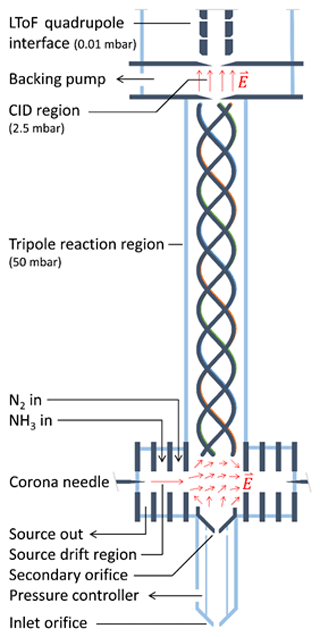Figure 1Schematic drawing of the ${\mathrm{NH}}_{\mathrm{4}}^{+}$ CIMS.

Reagent ions are generated in a corona discharge region and are extracted using a source drift region as indicated by red arrows in Fig. 1. The reaction chamber uses a tripole electrode configuration and is operated at typical pressures between 50 and 70 mbar. Unlike many other PTR instruments, there is no axial electric field accelerating ions towards the exit of the reaction chamber. Therefore, the reaction time is exclusively determined by the flow velocity of the sampled gas in the axial direction, leading to a typical reaction time of 3 ms. The long time-of-flight (LToF) mass spectrometer with a mass resolution m∕Δm of up to 8000 allows for the separation of the components with the same nominal mass.

The first major instrument design change consists of replacing the single ion source with three ion sources, one active at a time. Currently, we use two sources: one for producing H3O+(H2O)n, ($n=\mathrm{0},\mathrm{1}$) reagent ions (as PTR-MS, called H3O+ mode) and another for producing ${\mathrm{NH}}_{\mathrm{4}}^{+}\mathrm{•}\left({\mathrm{H}}_{\mathrm{2}}\mathrm{O}{\right)}_{n}$, ($n=\mathrm{0},\mathrm{1},\mathrm{2}$) reagent ions (as ${\mathrm{NH}}_{\mathrm{4}}^{+}$ CIMS, called ${\mathrm{NH}}_{\mathrm{4}}^{+}$ mode). ${\mathrm{NH}}_{\mathrm{4}}^{+}\mathrm{•}\left({\mathrm{H}}_{\mathrm{2}}\mathrm{O}{\right)}_{n}$ ions are produced in the corona discharge ion source from NH3 and H2O. A constant flow (20 sccm) of ammonia and water vapour is added to the ion source region from the headspace of a solution of ammonium hydroxide in water. For our setup, the concentration of the ammonium hydroxide aqueous solution of approximately 10 % leads to an optimal ${\mathrm{NH}}_{\mathrm{4}}^{+}\mathrm{•}\left({\mathrm{H}}_{\mathrm{2}}\mathrm{O}{\right)}_{n}$ primary ion signal with moderate impurities (Fig. S4 in the Supplement). At smaller concentrations, excessive H3O+(H2O)n primary ions are produced, while at higher concentrations ${\mathrm{NH}}_{\mathrm{4}}^{+}\mathrm{•}\left({\mathrm{NH}}_{\mathrm{3}}\right)$ becomes more prominent. Figure 1 shows the instrument in the ${\mathrm{NH}}_{\mathrm{4}}^{+}$ mode with the active ion source on the left, while the two other ion sources (depicted as a single ion source on the right) are inactive. The innermost source drift plate of the active ion source and the innermost source drift plates of both inactive sources generate an electric field perpendicular to the tripole axis. In addition, another component of the electric field is generated parallel to the tripole axis by biasing the electric potential at the secondary orifice relative to the tripole offset potential. Figure 1 illustrates the resulting electric field in this transfer region. This geometry allows for effective ion guiding from the active ion source to the centre of the reaction tripole chamber. Compared to single-source designs, separate ion sources allow for faster switching between reagent ion species. As shown in Fig. S3, switching from the H3O+ mode to the ${\mathrm{NH}}_{\mathrm{4}}^{+}$ mode occurs within 1 min, while the reverse switching from the ${\mathrm{NH}}_{\mathrm{4}}^{+}$ mode to the H3O+ mode can be done within 2 min.

The second major design change consists of replacing the straight tripole electrode rods with a helix. Simulations of ion trajectories in the original tripole showed that ions are lost mostly by exiting the device through spaces between the rods, rather than by collisions with the rods themselves, probably due to inhomogeneous effective potentials generated by the tripole radio frequency (RF) fields (Breitenlechner et al., 2017). The helical structure effectively averages these inhomogeneities, increasing the ion transmission efficiency and therefore the overall instrument performance.

The instrument can be used for measurements of organic molecules in both the gas and particle phases. During particle-phase measurements, sampled air passes through a gas-phase denuder (Ionicon Analytik GmbH, Austria) that removes the gas-phase organics and then through a thermal desorption region heated to 180 that vaporizes the aerosol particles. For more details see the Supplement.

3 ${\mathrm{NH}}_{\mathrm{4}}^{+}$ CIMS instrument performance

Multiple reagent ions are observed in the mass spectrum of this instrument in the ${\mathrm{NH}}_{\mathrm{4}}^{+}$ mode, including ammonium–water clusters ${\mathrm{NH}}_{\mathrm{4}}^{+}\mathrm{•}\left({\mathrm{H}}_{\mathrm{2}}\mathrm{O}{\right)}_{n}$, ($n=\mathrm{0},\mathrm{1},\mathrm{2}$) and ammonium ammonia dimers ${\mathrm{NH}}_{\mathrm{4}}^{+}\mathrm{•}\left({\mathrm{NH}}_{\mathrm{3}}\right)$. Humidity of the sampled air only slightly affects the distribution of the reagent ions, as shown in Fig. S4. Most organic molecules are detected as ammonium–organic clusters ${\mathrm{NH}}_{\mathrm{4}}^{+}\mathrm{•}\mathrm{VOC}$ with a few exceptions for which protonated ions VOCH+ are also observed. The protonated ions could be produced through proton switching reaction from either H3O+(H2O)n or ${\mathrm{NH}}_{\mathrm{4}}^{+}$. However, for all of these molecules the intensity of the ammonia–organic cluster is at least 1 order of magnitude higher than the intensity of the corresponding protonated ion.

Table 1Sensitivities and detection limits of ${\mathrm{NH}}_{\mathrm{4}}^{+}$ CIMS for various VOC species, voltage (V50), and corresponding kinetic energy (KEcm 50) at which half of the ions have dissociated.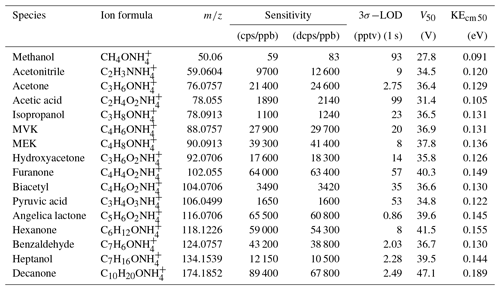A series of laboratory experiments were performed to obtain instrument sensitivities to various organic compounds as a function of relative humidity. Table 1 shows sensitivities to 16 compounds measured using a liquid calibration unit (LCU, Ionicon Analytik GmbH, Austria) at 10 % RH and 20. The LCU quantitatively evaporates aqueous standards into the gas stream. A total of 16 standards were prepared gravimetrically or volumetrically, depending on the compound, with aqueous volume mixing ratios of compounds ranging between 2 and 6 ppmv. An amount of 10 µL min−1 flow of each of these solutions was then evaporated into a humidified gas stream of synthetic air (9 slpm), resulting in calibration standards containing 1–2 ppbv of each calibrated component. In Table 1 we also present sensitivities calculated in duty-cycle-corrected counts per second per part per billion by volume (dcps ppbv−1, normalized to $m/z=\mathrm{100}$). The duty cycle correction compensates for the mass-dependent extraction efficiency into the time-of-flight mass spectrometer: dcps(i) = cps(i) $\cdot \sqrt{\mathrm{100}/{m}_{i}}$. The extraction frequency of the ToF was set at 14 kHz. Limits of detection are calculated for a 1 s integration time as 3 standard deviations of measured background divided by derived sensitivity. Sensitivity to each compound was measured at 10 %, 30 %, 50 %, and 70 % RH at 20. There is no strong correlation between the sensitivity to the calibrated compounds and their molecular weight (R2=0.35, Fig. S5).

Signals of ${\mathrm{NH}}_{\mathrm{4}}^{+}$–VOC clusters decrease as humidity of the sampled air increases, as shown in Fig. 2. Increased reaction time (3 ms) and elevated pressure (60 mbar) in the reaction chamber, compared to the conventional PTR-MS instruments (0.1 ms and 2.3 mbar, respectively), promote equilibrium between the forward and backward ligand-switching Reaction (R3). Hence, under humid conditions, excess water vapour favours formation of ammonium–water clusters, which in turn reduces the abundance of ammonium–organic clusters ${\mathrm{NH}}_{\mathrm{4}}^{+}\mathrm{•}\left(\mathrm{VOC}\right)$ and hence the overall instrument sensitivity to oxygenated VOCs (OVOCs). Humidity dependence of sensitivity does not show a strong correlation to cluster stability, as quantified by KE50 cm (R2=0.29, Fig. S6). In addition, correlation between humidity dependence of sensitivity and polarity of analyte molecules is relatively weak (R2=0.31).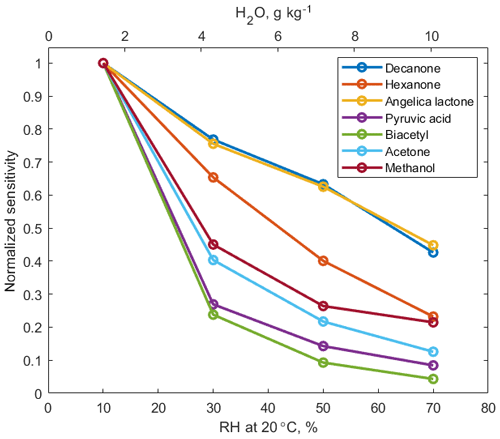Figure 2Humidity dependence curves for the normalized signals relative to the dryer conditions.

4 Collision-induced dissociation techniques for constraining sensitivity of the ${\mathrm{NH}}_{\mathrm{4}}^{+}$ CIMS

When the instrument operates in the ${\mathrm{NH}}_{\mathrm{4}}^{+}$ mode, organic molecules are detected almost entirely as ammonium–organic clusters. However, kinetic rate constants of ligand-switching Reaction (R3) from ammonium–water ions to an organic molecule have only been measured for very few analyte molecules. In addition, enhanced reaction time in the reaction chamber relative to conventional PTR-MS instruments increases probability of reverse ligand-switching reactions. Therefore, effective rate constants for both forward and backward Reaction (R3) are required for analytical estimation of the compound sensitivities. To avoid these complications, we constrain the instrument sensitivities to the detected compounds through an empirically based collision-induced dissociation (CID) technique similar to the one used by Lopez-Hilfiker et al. (2016) for constraining sensitivity of iodide adduct CIMS. This is accomplished by varying the voltage between the ionization region and vacuum region of the mass spectrometer (Fig. 1), which increases the electric field, while measuring intensities of detected peaks in the mass spectrum.

The increase in the collisional kinetic energy of the ammonium–organic clusters and air molecules leads to collision-induced dissociation of the clusters. For each analyte ion we determine the voltage value (V50) at which the peak intensity drops by 50 % relative to the intensity at the operational voltage value and calculate the ion kinetic energy corresponding to this voltage (KE50). Therefore, we can experimentally determine the electric field strength necessary to break each ammonium–organic cluster, which defines the stability of these clusters and hence the sensitivity of our instrument to analyte molecules. The value of EN (E is the electric field strength and N is the sample gas number density) is a suitable metric to characterize the motion of ions in the reaction chamber and kinematics of a chemical ionization reaction (Blake et al., 2006). The electric field strength E in a particular region of the reaction chamber depends on the voltage V applied in that region and the effective distance between electrodes d.

$\begin{array}{}\text{(1)}& E=\frac{V}{d}\end{array}$

Drift velocity of ions in the reaction chamber vd is determined by the electric field strength E and the ion mobility μ:

$\begin{array}{}\text{(2)}& {v}_{\mathrm{d}}=\mathit{\mu }E.\end{array}$

The ion mobility depends on reaction pressure and temperature:

$\begin{array}{}\text{(3)}& \mathit{\mu }={\mathit{\mu }}_{\mathrm{0}}\frac{\mathrm{1013}\phantom{\rule{0.125em}{0ex}}\mathrm{mbar}}{{p}_{\mathrm{r}}}\frac{{T}_{\mathrm{r}}}{\mathrm{273}\phantom{\rule{0.125em}{0ex}}\mathrm{K}},\end{array}$

where μ0 is the reduced mobility, which is estimated for each ion using its mass (Ehn et al., 2011), pr is pressure in the reaction chamber (mbar), and Tr is temperature in the reaction chamber (K). Further, we calculate mean kinetic energy of drifting ions KEion in the laboratory frame (Lindinger et al., 1998):

$\begin{array}{}\text{(4)}& {\mathrm{KE}}_{\mathrm{ion}}=\frac{\mathrm{3}}{\mathrm{2}}{k}_{\mathrm{B}}T+\frac{{M}_{\mathrm{buffer}}{v}_{\mathrm{d}}^{\mathrm{2}}}{\mathrm{2}}+\frac{{M}_{\mathrm{ion}}{v}_{\mathrm{d}}^{\mathrm{2}}}{\mathrm{2}},\end{array}$

where kB is the Boltzmann constant, and Mbuffer and Mion are the masses of the buffer molecule in the air and reagent ion, respectively. Finally, the kinetic energy of analyte ions in the centre of mass for ion–molecule collisions is given by (McFarland et al., 1973)

$\begin{array}{}\text{(5)}& {\mathrm{KE}}_{\mathrm{cm}}=\frac{{M}_{\mathrm{buffer}}}{{M}_{\mathrm{buffer}}+{M}_{\mathrm{ion}}}\left({\mathrm{KE}}_{\mathrm{ion}}-\frac{\mathrm{3}}{\mathrm{2}}{k}_{\mathrm{B}}T\right)+\frac{\mathrm{3}}{\mathrm{2}}{k}_{\mathrm{B}}T.\end{array}$

For each ammonium–organic cluster we measure V50 and from this calculate the corresponding kinetic energy at which half of the ions have dissociated (KEcm 50) using Eqs. (1)–(5). We show a set of de-clustering scans for eight organic molecules with different functional groups in Fig. 3. Intensities of all clusters follow similar sigmoidal shapes when the voltage is increased. Some clusters (i.e. small alcohols and heterocyclic compounds) are less stable and are dissociated at lower voltages while other clusters (i.e. large ketones) show higher stability. These scans can be obtained within 4 min by steadily increasing the voltage between the ionization region and vacuum region of the mass spectrometer (Fig. 1).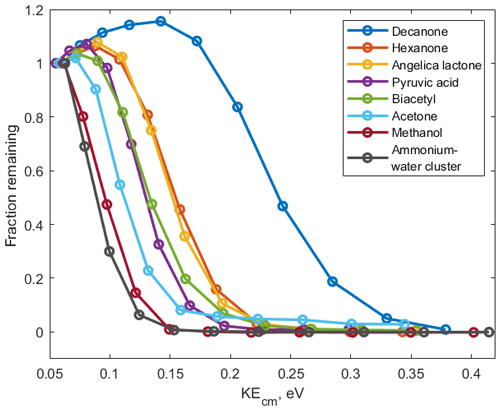Figure 3De-clustering scans of ammonium–organic clusters ${\mathrm{NH}}_{\mathrm{4}}^{+}$ (VOC) for calibrated components and ${\mathrm{NH}}_{\mathrm{4}}^{+}$ (H2O) reagent ions.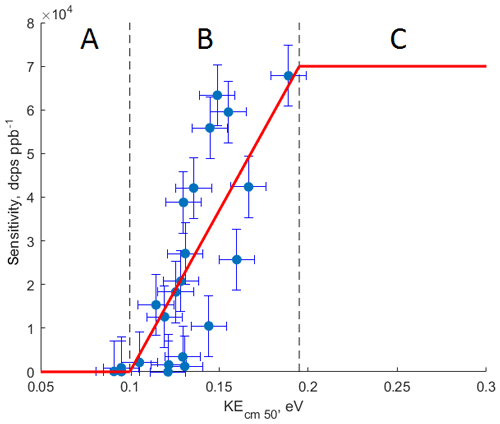Figure 4The relationship between calculated kinetic energy of the ammonium–organic clusters KEcm 50 and measured sensitivity for calibrated compounds. Molecules characterized by KEcm 50 smaller than 0.10 eV (region A) cannot be detected by ${\mathrm{NH}}_{\mathrm{4}}^{+}$ CIMS; for molecules characterized by KEcm 50 between 0.10 and 0.19 eV (region B) a linear relationship between KEcm 50 and measured sensitivity is observed; molecules characterized by KEcm 50 greater than 0.19 eV (region C) are detected at the “kinetic sensitivity”.

Figure 4 shows the relationship between the calculated kinetic energy KEcm 50 and measured sensitivity for 16 calibrated compounds at 10 % RH and 20. We observe a linear relationship (R2=0.61) between calculated KEcm 50 and measured sensitivity for calibrated VOCs. This linear relationship is observed for molecules with KEcm 50 in the range between 0.10 and 0.19 eV (region B in Fig. 4). Molecules characterized by collisional kinetic energies KEcm 50 smaller than that of the ammonium–water cluster (0.09 eV, region A in Fig. 4) will show no significant reaction rate since ligand-switching reactions between such molecules and ${\mathrm{NH}}_{\mathrm{4}}^{+}\mathrm{•}\left({\mathrm{H}}_{\mathrm{2}}\mathrm{O}\right)$ are endothermic. Conversely, the ligand-switching reaction rate cannot exceed the kinetic limit for ion–molecule collisions, and therefore there is also an upper limit of observed sensitivities. We calculate this limit by using experimentally determined pressure and reaction time in the reaction chamber and kinetic limit of ion–molecule reaction rate. We estimate the reaction time in the reaction chamber using the instrument sensitivity to specific compounds in the H3O+ mode. For polar compounds with proton affinity much higher than water (i.e. acetone), we can assume that reverse proton transfer reactions do not occur. In this case, the instrument sensitivity to those compounds is given by (Lindinger et al., 1998)

$\begin{array}{}\text{(6)}& \frac{i\left({\mathrm{RH}}^{+}\right)}{\left[R\right]}={i}_{\mathrm{primary}}\cdot k\cdot {t}_{\mathrm{react}}\cdot \frac{{p}_{\mathrm{react}}}{\mathrm{1013}\phantom{\rule{0.125em}{0ex}}\mathrm{mbar}},\end{array}$

where $\frac{i\left({\mathrm{RH}}^{+}\right)}{\left[R\right]}$ is the component sensitivity, iprimary is the primary ion current, k is the rate constant for the proton-transfer reaction (e.g. $k=\mathrm{3.6}×{\mathrm{10}}^{-\mathrm{9}}$ cm3 s−1 for acetone; Cappellin et al., 2012), and treact and preact are the reaction time and pressure in the reaction chamber, respectively. By measuring the instrument sensitivity to acetone in the H3O+ mode, we estimate treact to be 3 ms. In our case, the instrument sensitivity cannot exceed 70 000 dcps ppb−1, which is in agreement with the highest sensitivity measured for calibrated compounds. Therefore, we assume that all components with KEcm 50 greater than 0.19 eV (region C in Fig. 4) will be detected at this “kinetic sensitivity”. As shown in Fig. 2, the sensitivity of ${\mathrm{NH}}_{\mathrm{4}}^{+}$ CIMS to many calibrated compounds is RH dependent; thus we observe that the relationship between the calibrated kinetic energy KEcm 50 and the measured sensitivity also depends on the humidity of the sampled air (Fig. S7). Therefore, the values of the collisional limit and other calculated sensitivities reported herein are unique to the instrument setup (i.e. pressures and voltages in the reaction chamber) and vary with the humidity of the sampled air.

5 Application to secondary organic aerosols

To demonstrate the application of the procedure described above, we performed a series of laboratory chamber experiments. A complex mixture of organic compounds in both gas and particle phases was generated by the oxidation of 3-methylcatechol (C7H8O2), a second-generation oxidation product of toluene and other anthropogenic aromatics, by hydroxyl (OH) radicals in an environmental chamber. Details of the chamber operations are given by Hunter et al. (2014), so we include only a brief description here. Photochemical oxidation occurred in a 7.5 m3 temperature-controlled Teflon chamber by OH radicals generated through the photolysis of nitrous acid (HONO). In the experiment described here, 65 ppbv of 3-methylcatechol (Sigma-Aldrich, 98 % purity) was injected in the chamber and further oxidized in the presence of ammonium nitrate seed aerosol at 20 and low humidity (3 % RH). Secondary organic aerosol particles produced in this experiment were detected using an Aerodyne aerosol mass spectrometer (AMS; DeCarlo et al., 2006) and the described CIMS instrument operating in both the H3O+ and ${\mathrm{NH}}_{\mathrm{4}}^{+}$ modes, equipped with the thermal desorption unit described above. High-resolution mass spectra of 3-methylcatechol oxidation products derived in the ${\mathrm{NH}}_{\mathrm{4}}^{+}$-mode in the gas and particle phases are given in Fig. S8. In this experiment, we identified 202 peaks in the ${\mathrm{NH}}_{\mathrm{4}}^{+}$ mode mass spectra and grouped them based on the calculated KEcm 50 as shown in Fig. 5. Among those 202 $\mathrm{OC}\mathrm{•}{\mathrm{NH}}_{\mathrm{4}}^{+}$ peaks, 125 analyte formulas were also detected as OCH+ in the H3O+ mode. We plot the relationship between the detected signals in both modes of our instrument in Fig. 6. We use the de-clustering technique described above to calculate volume mixing ratios of organic molecules detected as ammonium–organic clusters in the ${\mathrm{NH}}_{\mathrm{4}}^{+}$ mode. In the H3O+ mode, we apply the calibrated acetone sensitivity to calculate volume mixing ratios of OVOCs. Breitenlechner et al. (2017) showed that due to the enhanced reaction time and the increased pressure in the reaction chamber the equilibrium between the forward and reverse proton reactions can be achieved. Hence, many compounds require careful calibration over a broad humidity range. Since PTR3 has the highest detected sensitivity to ketones, we use the acetone sensitivity to calculate the lower-limit concentration of OVOCs. Volume mixing ratios of organic compounds detected by both modes are in excellent agreement with a slope of 0.94 as shown in Fig. 6 (R2=0.78). In addition to 125 peaks measured by both modes, there are peaks that are detected solely by either the H3O+ or ${\mathrm{NH}}_{\mathrm{4}}^{+}$ mode. In Fig. 7, we plot 34 identified CxHyOzH+ peaks detected by the H3O+ mode and 17 identified ${\mathrm{C}}_{x}{\mathrm{H}}_{y}{\mathrm{O}}_{z}\mathrm{•}{\mathrm{NH}}_{\mathrm{4}}^{+}$ peaks detected by the ${\mathrm{NH}}_{\mathrm{4}}^{+}$ mode on the carbon number-oxidation state diagram. Two modes cover different areas on this diagram: while the ${\mathrm{NH}}_{\mathrm{4}}^{+}$ CIMS is able to detect larger and more functionalized molecules, PTR-MS is better at detection of smaller organic compounds (some of them can be formed as a result of fragmentation during ionization). Hence, the two modes complement each other and allow for the detection and quantification of a broader range of oxidized organic molecules. Similar observations about the selectivity of ${\mathrm{NH}}_{\mathrm{4}}^{+}$ CIMS and PTR-MS have been reported in the previous studies. Aljawhary et al. (2013) showed that H3O+(H2O)n primary ions are more selective to the detection of less oxidized water-soluble organic compounds (WSOCs) extracted from alpha-pinene SOA comparing to acetate CH3C(O)O and iodide water clusters I(H2O)n used as primary ions. Zhao et al. (2017) demonstrated that multiple positive reagent ions (${\mathrm{NH}}_{\mathrm{4}}^{+}$, Li+, Na+, K+) have higher selectivity for a wide range of highly oxygenated organics with higher molecular weights formed from ozonolysis of alpha-pinene, while negative reagent ions (I and ${\mathrm{NO}}_{\mathrm{3}}^{-}$) are more selective towards smaller species (e.g. CH2O2, CH2O3, C2H2O3, and C2H4O3).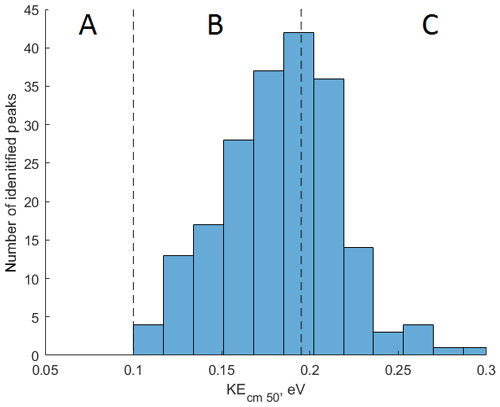Figure 5Application of the collision-induced dissociation techniques for measurement of SOA composition produced during photooxidation of 3-methylcatechol in a laboratory experiment. A total of 202 peaks are detected in ${\mathrm{NH}}_{\mathrm{4}}^{+}$ mode and binned based on their KEcm 50. Molecules with KEcm 50 smaller than 0.10 eV cannot be detected by ${\mathrm{NH}}_{\mathrm{4}}^{+}$ CIMS (region A); sensitivities of molecules characterized by KEcm 50 between 0.10 and 0.19 eV (region B) can be calculated using the linear fit presented in Fig. 4; molecules with KEcm 50 greater than 0.19 eV are detected at the “kinetic sensitivity” (region C).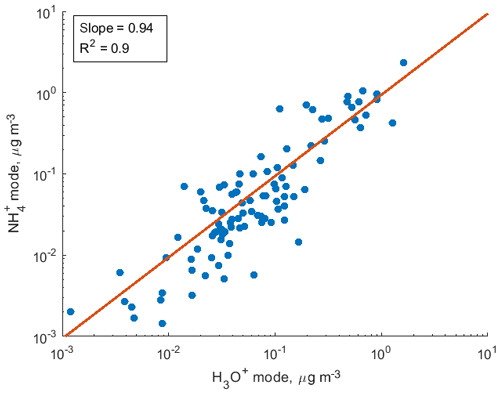Figure 6Comparison of volume mixing ratios of SOA components detected by the CIMS instrument in both H3O+ and ${\mathrm{NH}}_{\mathrm{4}}^{+}$ modes in the photooxidation experiment of 3-methylcatechol.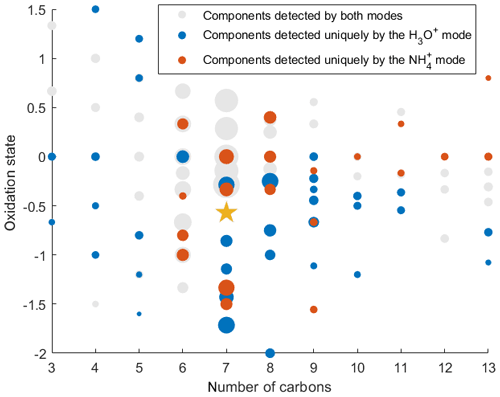Figure 7Identified SOA components detected in both H3O+ and ${\mathrm{NH}}_{\mathrm{4}}^{+}$ modes (125 peaks), uniquely in the H3O+ mode (34 peaks), and uniquely in ${\mathrm{NH}}_{\mathrm{4}}^{+}$ mode (17 peaks) plotted on the nC-${\stackrel{\mathrm{‾}}{\mathrm{OS}}}_{\mathrm{C}}$ diagram. The gold star corresponds to the precursor of the photooxidation experiment, 3-methylcatechol. The size of the dots is proportional to the logarithm of the volume mixing ratio of each compound produced at the end of the experiment.

Figure S9 shows a comparison between the total mass loading of all organic components measured by the AMS with the sum of masses of all organic compounds measured by our instrument in both H3O+ and ${\mathrm{NH}}_{\mathrm{4}}^{+}$ modes. The sum of signals of all components detected in the ${\mathrm{NH}}_{\mathrm{4}}^{+}$ mode account for 65 % of the total aerosol organic mass measured by the AMS as shown in Fig. 8. This discrepancy can be explained by a combination of the following factors: (1) uncertainties in the sensitivities obtained using the presented technique and in the AMS measurements; (2) thermal fragmentation of organic molecules in the thermal desorption unit, which leads to lower observed masses in the mass spectrum; (3) low ${\mathrm{NH}}_{\mathrm{4}}^{+}$ CIMS sensitivity to certain compounds of organic aerosols if ligand-switching reactions between these molecules and ammonium–water clusters are endothermic (e.g. small organic acids); and (4) wall losses of less volatile organic molecules in the ${\mathrm{NH}}_{\mathrm{4}}^{+}$ CIMS inlet. Although the ${\mathrm{NH}}_{\mathrm{4}}^{+}$ CIMS does not detect all organic compounds to explain the total organic mass measured by the AMS, it gives valuable insight into the composition of SOA as shown in Fig. 8.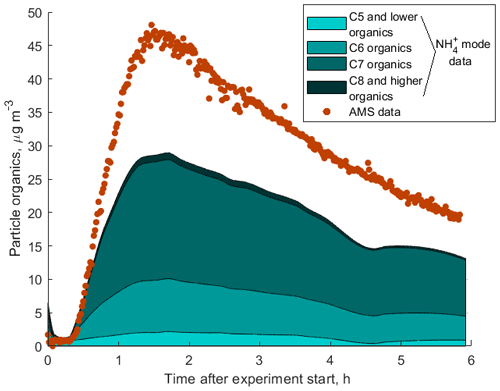Figure 8SOA produced during photooxidation of 3-methylcatechol in a laboratory experiment. The total organic aerosol mass is measured by an AMS. OVOCs detected by NH4 CIMS are binned in four groups.

6 Conclusions

In this study, a new CIMS instrument is described based on the recently introduced PTR3. The instrument can be operated in both ${\mathrm{NH}}_{\mathrm{4}}^{+}$ and H3O+ modes as ${\mathrm{NH}}_{\mathrm{4}}^{+}$ CIMS and PTR-MS, respectively, while switching between the two modes can be done within 2 min. Compared to the H3O+ mode, the ${\mathrm{NH}}_{\mathrm{4}}^{+}$ mode is able to detect more functionalized and larger organic molecules. In the ${\mathrm{NH}}_{\mathrm{4}}^{+}$ mode, the instrument has sensitivities in the range of 80–65 000 dcps ppbv−1 and detection limits in the range of 1.5–60 pptv for a 1 s integration time (2σ). We present a procedure based on collision-induced dissociation that allows us to estimate the stability of detected ammonium–organic clusters and therefore to constrain the sensitivities of hundreds of compounds detected by the ${\mathrm{NH}}_{\mathrm{4}}^{+}$ mode of the new instrument without their direct calibration within several minutes.

Data availability
Data availability.

Data used within this work are available upon request. Please email Alexander Zaytsev (zaytsev@g.harvard.edu).

Supplement
Supplement.

Author contributions
Author contributions.

MB and AZ designed and built the CIMS instrument. AZ and MB developed the methodology with contributions from ARC and FNK. AZ, ARC, and MB performed the laboratory experiments. AZ and MB provided data and analysis for the CIMS instrument. CYL and JCR provided data and analysis for the AMS instrument. AZ prepared the paper with contributions from all co-authors.

Competing interests
Competing interests.

The authors declare that they have no conflict of interest.

Acknowledgements
Acknowledgements.

This work was supported by the Harvard Global Institute and the NSF award AGS-1638672. Martin Breitenlechner acknowledges support from the Austrian science fund (FWF), grant J-3900. Abigail R. Koss acknowledges support from the Dreyfus Postdoctoral Program.

Review statement
Review statement.

This paper was edited by Keding Lu and reviewed by two anonymous referees.

References

Aljawhary, D., Lee, A. K. Y., and Abbatt, J. P. D.: High-resolution chemical ionization mass spectrometry (ToF-CIMS): application to study SOA composition and processing, Atmos. Meas. Tech., 6, 3211–3224, https://doi.org/10.5194/amt-6-3211-2013, 2013.

Atkinson R.: Atmospheric chemistry of VOCs and NOx, Atmos. Environ., 34, 2063–2101, 2000.

Berresheim, H., Elste, T., Plass-Dülmer, C., Eisele, F. L., and Tanner, D. J.: Chemical ionization mass spectrometer for long-term measurements of atmospheric OH and H2SO4, Int. J. Mass Spectrom., 202, 91–109, https://doi.org/10.1016/S1387-3806(00)00233-5, 2000.

Bertram, T. H., Kimmel, J. R., Crisp, T. A., Ryder, O. S., Yatavelli, R. L. N., Thornton, J. A., Cubison, M. J., Gonin, M., and Worsnop, D. R.: A field-deployable, chemical ionization time-of-flight mass spectrometer, Atmos. Meas. Tech., 4, 1471–1479, https://doi.org/10.5194/amt-4-1471-2011, 2011.

Blake, R. S., Whyte, C., Hughes, C. O., Ellis, A. M., and Monks, P. S.: Demonstration of Proton-Transfer Reaction Time-of-Flight Mass Spectrometry for Real-Time Analysis of Trace Volatile Organic Compounds, Anal. Chem., 76, 3841–3845, https://doi.org/10.1021/ac0498260, 2004.

Blake, R. S., Wyche, K. P., Ellis, A. M., and Monks, P. S.: Chemical ionization reaction time-of-flight mass spectrometry: Multi-reagent analysis for determination of trace gas composition, Int. J. Mass Spectrom., 254, 85–93, https://doi.org/10.1016/j.ijms.2006.05.021, 2006.

Breitenlechner, M., Fischer, M., Hainer, M., Heinritzi, M., Curtius, M., and Hansel, A.: PTR3: An instrument for Studying the Lifecycle of Reactive Organic Carbon in the Atmosphere, Anal. Chem., 89, 5824–5831, https://doi.org/10.1021/acs.analchem.6b05110, 2017.

Cappellin, L., Karl,T., Probst, M., Ismailova, O., Winkler, P. M., Soukoulis, C., Aprea, E., Märk, T. D., Gasperi, F., and Biasioli, F.: On Quantitative Determination of Volatile Organic Compound Concentrations Using Proton Transfer Reaction Time-of-Flight Mass Spectrometry, Environ. Sci. Technol., 46, 2283–2290, https://doi.org/10.1021/es203985t, 2012.

Crounse, J. D., McKinney, K. A., Kwan, A. J., and Wennberg, P. O.: Measurement of Gas-Phase Hydroperoxides by Chemical Ionization Mass Spectrometry, Anal. Chem., 78, 6726–6732, https://doi.org/10.1021/ac0604235, 2006.

DeCarlo, P. F., Kimmel, J. R., Trimborn, A., Northway, M. J., Jayne, J. T., Aiken, A. C., Gonin, M., Fuhrer, K., Horvath, T., Docherty, K. S., Worsnop, D. R., and Jimenez, J. L.: Field-Deployable, High-Resolution, Time-of-Flight Aerosol Mass Spectrometer, Anal. Chem., 78, 8281–8289, https://doi.org/10.1021/ac061249n, 2006.

Ehn, M., Junninen, H., Schobesberger, S., Manninen, H. E., Franchin, A., Sipilä, M., Petäjä, T., Kerminen, V.-M., Tammet, H., Mirme, A., Mirme, S., Hõrrak, U., Kulmala, M., and Worsnop, D. R.: An Instrumental Comparison of Mobility and Mass Measurements of Atmospheric Small Ions, Aerosol Sci. Tech., 45, 522–532, https://doi.org/10.1080/02786826.2010.547890, 2011.

Eichler, P., Müller, M., D'Anna, B., and Wisthaler, A.: A novel inlet system for online chemical analysis of semi-volatile submicron particulate matter, Atmos. Meas. Tech., 8, 1353–1360, https://doi.org/10.5194/amt-8-1353-2015, 2015.

Jokinen, T., Sipilä, M., Junninen, H., Ehn, M., Lönn, G., Hakala, J., Petäjä, T., Mauldin III, R. L., Kulmala, M., and Worsnop, D. R.: Atmospheric sulphuric acid and neutral cluster measurements using CI-APi-TOF, Atmos. Chem. Phys., 12, 4117–4125, https://doi.org/10.5194/acp-12-4117-2012, 2012.

Hansel, A., Jordan, A., Holzinger, R., Prazeller, P., Vogel, W., and Lindinger, W.: Proton transfer reaction mass spectrometry: on-line trace gas analysis at the ppb level, Int. J. Mass Spectrom. Ion Process., 149–150, 609–619, https://doi.org/10.1016/0168-1176(95)04294-U, 1995.

Hansel, A., Scholz, W., Mentler, B., Fischer L., and Berndt, T.: Detection of RO2 radicals and other products from cyclohexene ozonolysis with ${\mathrm{NH}}_{\mathrm{4}}^{+}$ and acetate ionization mass spectrometry, Atmos. Env., 186, 248–255, https://doi.org/10.1016/j.atmosenv.2018.04.023, 2018.

Hellen, H., Dommen, J., Metzger, A., Gascho, A., Duplissy, J., Tritscher, T., Prevot, A. S. H., and Baltensperger, U.: Using Proton Transfer Reaction Mass Spectrometry for Online Analysis of Secondary Organic Aerosols, Environ. Sci. Technol., 42, 7347–7353, https://doi.org/10.1021/es801279m, 2008.

Hunter, J. F., Carrasquillo, A. J., Daumit, K. E., and Kroll, J. H.: Secondary Organic Aerosol Formation from Acyclic, Monocyclic, and Polycyclic Alkanes, Environ. Sci. Technol., 48, 10227–10234, https://doi.org/10.1021/es502674s, 2014

Hyttinen, N., Otkjær, R. V., Iyer, S., Kjaergaard, H. G., Rissanen, M. P., Wennberg, P. O., and Kurtén, T.: Computational Comparison of Different Reagent Ions in the Chemical Ionization of Oxidized Multifunctional Compounds, J. Phys. Chem A, 122, 269–279, https://doi.org/10.1021/acs.jpca.7b10015, 2017.

Krechmer, J., Lopez-Hilfiker, F., Koss, A., Hutterli, M., Stoermer, C., Deming, B., Kimmel, J., Warneke, C., Holzinger, R., Jayne, J., Worsnop, D., Fuhrer, K., Gonin, M., and de Gouw, J.: Evaluation of a New Vocus Reagent-Ion Source and Focusing Ion-Molecule Reactor for use in Proton-Transfer-Reaction Mass Spectrometry, Anal. Chem., 90, 12011–12018, https://doi.org/10.1021/acs.analchem.8b02641, 2018.

Kroll, J. H., Donahue, N. M., Jimenez, J. L., Kessler, S. H., Canagaratna, M. R., Wilson, K. R., Altieri, K. E., Mazzoleni, L. R., Wozniak, A. S., Bluhm, H., Mysak, E. R., Smith, J. D., Kolb, C. E., and Worsnop, D. R.: Carbon oxidation state as a metric for describing the chemistry of atmospheric organic aerosol, Nat. Chem., 3, 133–139, https://doi.org/10.1038/NCHEM.948, 2011.

Lee, B. H., Lopez-Hilfiker, F. D., Mohr, C., Kurten, T., Worsnop, D. R., and Thornton, J.: An iodide-adduct high-resolution time-of-flight chemical-ionization mass spectrometer: Application to atmospheric organic and inorganic compounds, Environ. Sci. Technol., 48, 6309–6317, https://doi.org/10.1021/es500362a, 2014.

Lindinger, W., Hansel, A., and Jordan, A.: On-line monitoring of volatile organic compounds at pptv levels by means of proton-transfer-reaction mass spectrometry (PTR-MS) medical applications, food control and environmental research, Int. J. Mass Spectrom., 173, 191–241, https://doi.org/10.1016/S0168-1176(97)00281-4, 1998.

Lopez-Hilfiker, F. D., Mohr, C., Ehn, M., Rubach, F., Kleist, E., Wildt, J., Mentel, Th. F., Lutz, A., Hallquist, M., Worsnop, D., and Thornton, J. A.: A novel method for online analysis of gas and particle composition: description and evaluation of a Filter Inlet for Gases and AEROsols (FIGAERO), Atmos. Meas. Tech., 7, 983–1001, https://doi.org/10.5194/amt-7-983-2014, 2014.

Lopez-Hilfiker, F. D., Iyer, S., Mohr, C., Lee, B. H., D'Ambro, E. L., Kurtén, T., and Thornton, J. A.: Constraining the sensitivity of iodide adduct chemical ionization mass spectrometry to multifunctional organic molecules using the collision limit and thermodynamic stability of iodide ion adducts, Atmos. Meas. Tech., 9, 1505–1512, https://doi.org/10.5194/amt-9-1505-2016, 2016.

McFarland, M., Albritton, D. L., Fehsenfeld, F. C., Ferguson, E. E., and Schmeltekopf, A. L.: Flow-drift technique for ion mobility and ion-molecule reaction rate constant measurements, II, Positive ion reactions of N+, O+, and with O2 and O+ with N2 from thermal to ∼2 eV, J. Chem. Phys., 59, 6620, https://doi.org/10.1063/1.1680042, 1973.

Shen, C., Li, J., Han, H., Wang, H., Jiang, H., and Chu, Y.: Triacetone triperoxide detection using low reduced-field proton transfer reaction mass spectrometer, Int. J. Mass Spectrom, 285, 100–103, https://doi.org/10.1016/j.ijms.2009.04.007, 2009.

Shrivastava, M., Kappa, C. D., Fan, J., Goldstein, A. H., Guenther, A. B., Jimenez, J. L., Kuang, C., Laskin, A., Martin, S. T., Ng, N. L., Petaja, T., Pierce, J. R., Rasch, P. J., Roldin, P., Seinfeld, J. H., Shilling, J., Smith, J. N., Thornton, J. A., Volkamer, R., Wang, J., Worsnop, D. R., Zaveri, R. A., Zelenyuk, A., and Zhang, Q.: Review of Recent Advances in Understanding Secondary Organic Aerosol for Earth System Modeling, Rev. Geophys., 55, 509–559, https://doi.org/10.1002/2016RG000540, 2017.

Yuan, B., Koss, A. R., Warneke, C., Coggon, M., Sekimoto, K., and de Gouw, J. A.: Proton-Transfer-Reaction Mass Spectrometry: Applications in Atmospheric Sciences, Chem. Rev., 117, 13187–13229, https://doi.org/10.1021/acs.chemrev.7b00325, 2017.

Zhao, Y., Chan, J. K., Lopez-Hilfiker, F. D., McKeown, M. A., D'Ambro, E. L., Slowik, J. G., Riffell, J. A., and Thornton, J. A.: An electrospray chemical ionization source for real-time measurement of atmospheric organic and inorganic vapors, Atmos. Meas. Tech., 10, 3609–3625, https://doi.org/10.5194/amt-10-3609-2017, 2017.